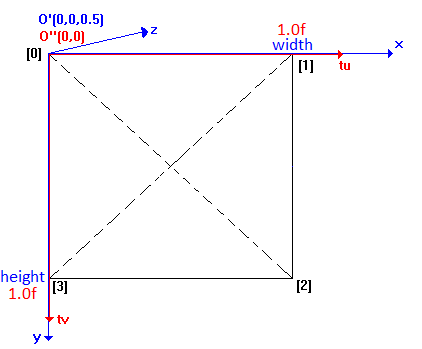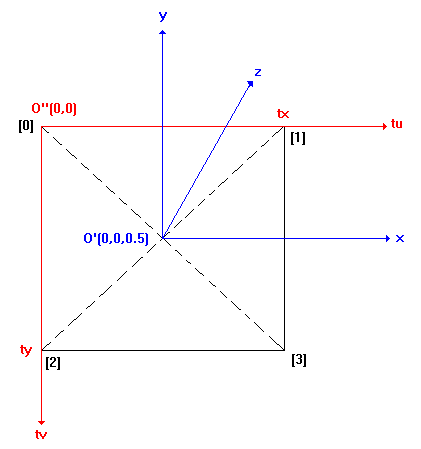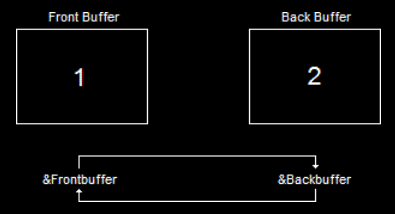VDJPedia

Plugins_SDKv8_VideoExplanations

Video Effects & Transitions in VirtualDJ 8 (How To in Direct3D 9)

1) The first step in order to use Direct3D9 is to have to get a pointer to IDirect3DDevice9 that is defined by the VirtualDJ sofware. This pointer is available in the VirtualDJ video plugins SDK by using the following function:

IDirect3DDevice9 *D3DDevice;
void *device = NULL;
HRESULT hr;

hr = GetDevice(VdjVideoEngineDirectX9, &device);
if(hr!=S_OK || device==NULL) return S_FALSE;
D3DDevice = reinterpret_cast<IDirect3DDevice9*>(device);

Each time you want to use a Direct3D functions, just use:

D3DDevice->xxxxxxxxxxx

2) A vertex is a dot in the space defined by the following structure
(NB: one vertex, two vertices)

struct TVertex
{
struct {FLOAT x,y,z;} position; // The 3D position (coordinates) for the vertex
DWORD color; // The vertex color
FLOAT tu,tv; // The texture coordinates
};

3D models in Direct3D are based on triangles:
To define a triangle, you need 3 Vertices: vertices, vertices, vertices
To define a square, you need 4 Vertices: vertices, vertices, vertices, vertices and in other words you need 2 triangles : {vertices, vertices, vertices} and {vertices, vertices, vertices}

SDK 8 | SDK 7 (and previous):It returns the following data fo the pointer Vertex:
(width and height are available in the VirtualDJ video plugins SDK)
(For information: D3DCOLOR_RGBA(255,255,255,255) = 4294967295)
Vertex.position.x=0.0f * (float) width;
Vertex.position.y=0.0f * (float) height;
Vertex.position.z=0.5f;
Vertex.color=D3DCOLOR_RGBA(255,255,255,255);
Vertex.tu=0.0f;
Vertex.tv=0.0f;

Vertex.position.x= 1.0f * (float) width;
Vertex.position.y=0.0f * (float) height;
Vertex.position.z=0.5f;
Vertex.color=D3DCOLOR_RGBA(255,255,255,255);
Vertex.tu=1.0f;
Vertex.tv=0.0f;

Vertex.position.x=1.0f * (float) width;
Vertex.position.y=1.0f * (float) height;
Vertex.position.z=0.5f;
Vertex.color=D3DCOLOR_RGBA(255,255,255,255);
Vertex.tu=1.0f;
Vertex.tv=1.0f;

Vertex.position.x=0.0f * (float) width;;
Vertex.position.y=1.0f * (float) height;
Vertex.position.z=0.5f;
Vertex.color=D3DCOLOR_RGBA(255,255,255,255);
Vertex.tu=0.0f;
Vertex.tv=1.0f;

3) Texturing:

"IDirect3DTexture9 *texture" is the current video texture

Then you apply the texture (video,bitmap,...) on this square with the following function:

D3DDevice->SetTexture(0,texture);

NB: in this case "0" means the first stage of the video

For a Video FX:
IDirect3DTexture9 *texture;
TVertex *Vertex;
HRESULT hr;

hr = GetTexture(VdjVideoEngineDirectX9, (void **) &texture, &Vertex);

4) Renderering:

return S_FALSE; will automatically render the video for you

if you want to render by yourself, you can use for example the following function:
D3DDevice->DrawPrimitiveUP(D3DPT_TRIANGLESTRIP,2,(LPVOID)Vertices,sizeof(Vertices));
and in this case, use return S_OK;

Some source code examples:

#ifndef SAFE_RELEASE
#define SAFE_RELEASE(x) { if (x!=NULL) { x->Release(); x=NULL; } }
#endif

{
D3DDevice = NULL;
g_font = NULL;
return S_OK;
}

HRESULT VDJ_API CMyPlugin8::OnDeviceInit()
{
GetDevice(VdjVideoEngineDirectX9, (void **)&D3DDevice);
D3DXCreateFont(D3DDevice, 22, 0, FW_NORMAL, 1, false, DEFAULT_CHARSET, OUT_DEFAULT_PRECIS, ANTIALIASED_QUALITY, DEFAULT_PITCH | FF_DONTCARE, "Arial", &g_font);
return S_OK;
}

HRESULT VDJ_API CMyPlugin8::OnDraw()
{
if (!g_font || !D3DDevice)
return S_FALSE;

DrawDeck();

RECT font_rect;
SetRect(&font_rect, 0, 0, width, height);
g_font->DrawText(NULL, fps_string, -1, &font_rect, DT_LEFT | DT_NOCLIP, 0xFFFFFFFF);

return S_OK;
}

HRESULT VDJ_API CMyPlugin8::OnDeviceClose()
{
SAFE_RELEASE(g_font);
D3DDevice = NULL;
return S_OK;
}

If you're wanting to draw the default VDJ video with modified vertices, then do something like this:

TVertex *v;
IDirect3DTexture9 *t;
GetTexture(VdjVideoEngineAny, (void**)&t, &v);
// modify vertices here
DrawDeck();

Finally if you're wanting to do something with your own vertices then have a play with something like this:

#define D3DFVF_TLVERTEX3D (D3DFVF_XYZ|D3DFVF_DIFFUSE|D3DFVF_TEX1)

HRESULT VDJ_API CMyPlugin8::OnDeviceInit()
{
GetDevice(VdjVideoEngineAny, (void**)&device);
if (FAILED(device->CreateVertexBuffer(4 * sizeof(TVertex), D3DUSAGE_WRITEONLY, D3DFVF_TLVERTEX3D, D3DPOOL_DEFAULT, &vertexBuffer, NULL)))
return E_FAIL;
return S_OK;
}

HRESULT VDJ_API CMyPlugin8::OnDeviceClose()
{
SAFE_RELEASE(vertexBuffer);
device = NULL;
return S_OK;
}

bool CMyPlugin8::UpdateVertices()
{
if (FAILED(vertexBuffer->Lock(0, 0, (void**)&vertices, 0)))
{
vertexBuffer->Release();
vertexBuffer = NULL;
return false;
}
vertices.position.x = 0.0f;
vertices.position.y = 0.0f;
vertices.position.z = 0.5f;
vertices.color = D3DCOLOR_RGBA(255, 255, 255, 255);
vertices.tu = 0.f;
vertices.tv = 0.f;
vertices.position.x = (float)width;
vertices.position.y = 0.0f;
vertices.position.z = 0.5f;
vertices.color = D3DCOLOR_RGBA(255, 255, 255, 255);
vertices.tu = 1.f;
vertices.tv = 0.f;
vertices.position.x = (float)width;
vertices.position.y = (float)height;
vertices.position.z = 0.5f;
vertices.color = D3DCOLOR_RGBA(255, 255, 255, 255);
vertices.tu = 1.f;
vertices.tv = 1.f;
vertices.position.x = 0.0f;
vertices.position.y = (float)height;
vertices.position.z = 0.5f;
vertices.color = D3DCOLOR_RGBA(255, 255, 255, 255);
vertices.tu = 0.f;
vertices.tv = 1.f;
vertexBuffer->Unlock();
return true;
}

HRESULT VDJ_API CMyPlugin8::OnDraw()
{
if (!device)
return S_FALSE;

TVertex *v;
IDirect3DTexture9 *t;
GetTexture(VdjVideoEngineAny, (void**)&t, &v);

UpdateVertices();

device->SetTexture(0, t);

device->SetStreamSource(0, vertexBuffer, 0, sizeof(TVertex));
device->SetFVF(D3DFVF_TLVERTEX3D);
device->DrawPrimitiveUP(D3DPT_TRIANGLEFAN, 2, (LPVOID)vertices, sizeof(vertices));

return S_OK;
}

Direct3D approaches: